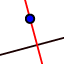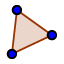# Right-angle-Hypotenuse-Side

## RHS Explained

If the hypotenuse and one side of a right-angled triangle are congruent to the corresponding parts of another right triangle, the right-angled triangles are congruent. Remember during SSS we also experience that the triangle became a right-angle triangle when the sides were Pythagorean Triples. Read more about Pythagorean Triples by clicking here: We can always use the Pythagorean Theorem to work out the third side and the use the SSS-Approach to construct this. But, this is a special case of ASS that will be discussed in more detail under the section about problematic methods. (See the "Donkey Theorem" for more details) Below is an example how to construct this. If you change anything in the construction, just click on the arrows on the top right to restore the construction.

## Steps in Constructing RHS

Now you try to draw a triangle congruent to the previous one﻿ You need to draw a triangle with side AB=8cm, right angle CAB and hypotenuse of 10cm.  Try to do this in the "Applet" below

1. Useto draw segment AB and if you are requested to give the length type in 8
2. Useto draw a circle at point B and if requested to enter a radius type in 10
3. Useto draw a line perpendicular to line AB through point A. (Click on line AB and then point A.)
4. Useto place point C at the intersection of the perpendicular line and the circle
5. Useto draw triangle ABC